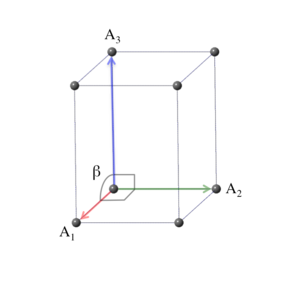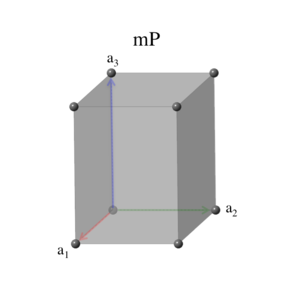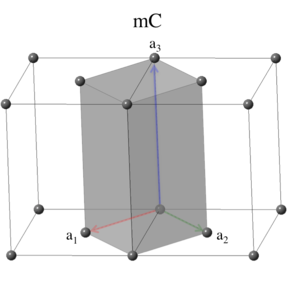# Encyclopedia of Crystallographic Prototypes

M. J. Mehl, D. Hicks, C. Toher, O. Levy, R. M. Hanson, G. L. W. Hart, and S. Curtarolo, The AFLOW Library of Crystallographic Prototypes: Part 1, Comp. Mat. Sci. 136, S1-S828 (2017). (doi=10.1016/j.commatsci.2017.01.017)
D. Hicks, M. J. Mehl, E. Gossett, C. Toher, O. Levy, R. M. Hanson, G. L. W. Hart, and S. Curtarolo, The AFLOW Library of Crystallographic Prototypes: Part 2, Comp. Mat. Sci. 161, S1-S1011 (2019). (doi=10.1016/j.commatsci.2018.10.043)

## The Monoclinic Crystal SystemIn the monoclinic crystal system, the conventional unit cell is defined by primitive vectors of arbitrary length, where one of the vectors is perpendicular to the other two. Modern convention chooses this vector to be the one with length $b$ (or unique axis $b$ in the literature), so that $\alpha = \gamma = \pi/2$ and $\beta \ne \pi/2$. Note that this orientation differs from that of Setyawan and Curtarolo who used an unique axis $a$ setting. Their angle $\alpha$ would be $\gamma$ in our notation. The conventional unit cell can be described by the vectors \begin{eqnarray} \mathbf{A}_1 & = & a \, \mathbf{\hat{x}} \nonumber \\ \mathbf{A}_2 & = & b \, \mathbf{\hat{y}} \nonumber \\ \mathbf{A}_3 & = & c \, \cos\beta \, \mathbf{\hat{x}} + c \, \sin\beta \, \mathbf{\hat{z}}, \end{eqnarray} and the volume of a conventional unit cell is \begin{equation} V = a \, b \, c \, \sin\beta. \end{equation}

### Lattice 2: Simple MonoclinicThe simple monoclinic cell is identical to the conventional cell: \begin{eqnarray} \mathbf{a}_1 & = & a \, \mathbf{\hat{x}} \nonumber \\ \mathbf{a}_2 & = & b \, \mathbf{\hat{y}} \nonumber \\ \mathbf{a}_3 & = & c \, \cos\beta \, \mathbf{\hat{x}} + c \, \sin\beta \, \mathbf{\hat{z}}, \end{eqnarray} and the cell volume is just \begin{equation} V = a \, b \, c \, \sin\beta. \end{equation}
The space groups associated with the simple monoclinic lattice are \begin{array}{lll} 3. ~ \mbox{P2} & 4. ~ \mbox{P2$_{1}$} & 6. ~ \mbox{Pm} \\ 7. ~ \mbox{Pc} & 10. ~ \mbox{P2/m} & 11. ~ \mbox{P2$_{1}$/m} \\ 13. ~ \mbox{P2/c} & 14. ~ \mbox{P2$_{1}$/c} & \\ \end{array}

### Lattice 3: Base-Centered MonoclinicThe base-centered monoclinic lattice is in the same crystal system as the monoclinic lattice, but its periodicity allows an additional translation in the plane defined by $\mathbf{a}_1$ and $\mathbf{a}_2$. The primitive vectors for the base-centered monoclinic lattice can be written as \begin{eqnarray} \mathbf{a}_1 & = & \frac{a}{2} \, \mathbf{\hat{x}} - \frac{b}{2} \, \mathbf{\hat{y}} \nonumber \\ \mathbf{a}_2 & = & \frac{a}{2} \, \mathbf{\hat{x}} + \frac{b}{2} \, \mathbf{\hat{y}} \nonumber \\ \mathbf{a}_3 & = & c \, \cos\beta \, \mathbf{\hat{x}} + c \, \sin\beta \, \mathbf{\hat{z}}. \end{eqnarray} The volume of the base-centered monoclinic unit cell is \begin{equation} V = \left(\frac{1}{2}\right) \, a \, b \, c \, \sin\beta, \end{equation} which is half that of the conventional unit cell.
The space groups associated with the base-centered monoclinic lattice are \begin{array}{lll} 5. ~ \mbox{C2} & 8. ~ \mbox{Cm} & 9. ~ \mbox{Cc} \\ 12. ~ \mbox{C2/m} & 15. ~ \mbox{C2/c} & \\ \end{array} The labels for these space groups all begin with $\mbox{C}$, indicating the base-centered translation associated with these groups. The International Tables offer two representations of the base-centered monoclinic space groups, one for unique axis $b$ and one for unique axis $c$, where $\alpha \ne \pi/2$ and $\beta = \pi/2$. Space group 5 is then listed as B2 or C2 depending on this choice. Most authors ignore this distinction, as will we.